Originally published in the May/June 2014 issue of Java Magazine. Subscribe today.

## Part 2: Processing Data with Java SE 8 Streams

by Raoul-Gabriel Urma

Published May 2014

Combine advanced operations of the Stream API to express rich data processing queries.

In the first part of this series, you saw that streams let you process collections with database-like operations. As a refresher, the example in Listing 1 shows how to sum the values of only expensive transactions using the Stream API. We set up a pipeline of operations consisting of intermediate operations (`filter`, `map`) and a terminal operation (`reduce`), as illustrated in Figure 1.

```int sumExpensive =
transactions.stream()
.filter(t -> t.getValue() > 1000)
.map(Transaction::getValue)
.reduce(0, Integer::sum);
```

Listing 1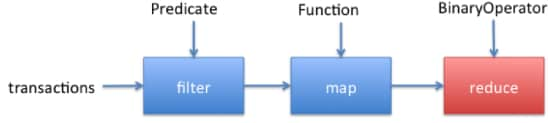Figure 1

However, the first part of this series didn’t investigate two operations:

• `flatMap`: An intermediate operation that lets you combine a “map” and a “flatten” operation
• `collect`: A terminal operation that takes as an argument various recipes (called collectors) for accumulating the elements of a stream into a summary result

These two operations are useful for expressing more-complex queries. For instance, you can combine `flatMap` and `collect` to produce a `Map` representing the number of occurrences of each character that appears in a stream of words, as shown in Listing 2. Don’t worry if this code seems overwhelming at first. The purpose of this article is to explain and explore these two operations in more detail.

```import static java.util.function.Function.identity;
import static java.util.stream.Collectors.*;

Stream<String> words = Stream.of("Java", "Magazine", "is",
"the", "best");

Map<String, Long> letterToCount =
words.map(w -> w.split(""))
.flatMap(Arrays::stream)
.collect(groupingBy(identity(), counting()));
```

Listing 2

The code in Listing 2 will produce the output shown in Listing 3. Awesome, isn’t it? Let’s get started and explore how the `flatMap` and `collect` operations work.

```[a:4, b:1, e:3, g:1, h:1, i:2, ..]
```

Listing 3

### flatMap Operation

 Using the Stream API and collectors, you can combine collectors together to create powerful queries, such as multilevel groupings.
Suppose you would like to find all unique words in a file. How would you go about it?

You might think that it is easy; we can use `Files.lines()`, which you saw in the previous article, because it can return a stream consisting of the lines of a file. We can then split each line into words using a `map()` operation and, finally, use the operation `distinct()` to remove duplicates. A first attempt could be the code shown in Listing 4.

```Files.lines(Paths.get("stuff.txt"))
.map(line -> line.split("\\s+")) // Stream<String[]>
.distinct() // Stream<String[]>
.forEach(System.out::println);
```

Listing 4

Unfortunately, this is not quite right. If you run this code, you will get puzzling output similar to this:

```[Ljava.lang.String;@7cca494b
[Ljava.lang.String;@7ba4f24f
…
```

Our first attempt is actually printing the `String` representation of several streams! What is happening? The problem with this approach is that the lambda passed to the `map` method returns an array of `String (String[])` for each line in the file. As a result, the stream returned by the `map` method is actually of type `Stream<String[]>`. What we really want is `Stream<String>` to represent a stream of words.

Luckily there’s a solution to this problem using the method `flatMap`. Let’s see step-by-step how to get to the right solution.

First, we need a stream of words instead of a stream of arrays. There’s a method called `Arrays .stream()` that takes an array and produces a stream. See the example shown in Listing 5.

```String[] arrayOfWords = {"Java", "Magazine"};
Stream<String> streamOfwords = Arrays.stream(arrayOfWords);
```

Listing 5

Let’s use it in our previous stream pipeline to see what happens (see Listing 6). The solution still doesn’t work. This is because we now end up with a list of streams of streams (more precisely a `Stream<Stream<String>>`). Indeed, we first convert each line into an array of words, and then convert each array into a separate stream using the method `Arrays.stream()`.

```Files.lines(Paths.get("stuff.txt"))
.map(line -> line.split("\\s+")) // Stream<String[]>
.map(Arrays::stream) // Stream<Stream<String>>
.distinct() // Stream<Stream<String>>
.forEach(System.out::println);
```

Listing 6

We can fix this problem by using a `flatMap`, as shown in Listing 7. Using the `flatMap` method has the effect of replacing each generated array not by a stream but by the contents of that stream. In other words, all the separate streams that were generated when using `map(Arrays::stream)` get amalgamated or “flattened” into one single stream. Figure 2 illustrates the effect of using the `flatMap` method.

```Files.lines(Paths.get("stuff.txt"))
.map(line -> line.split("\\s+")) // Stream<String[]>
.flatMap(Arrays::stream) // Stream<String>
.distinct() // Stream<String>
.forEach(System.out::println);
```

Listing 7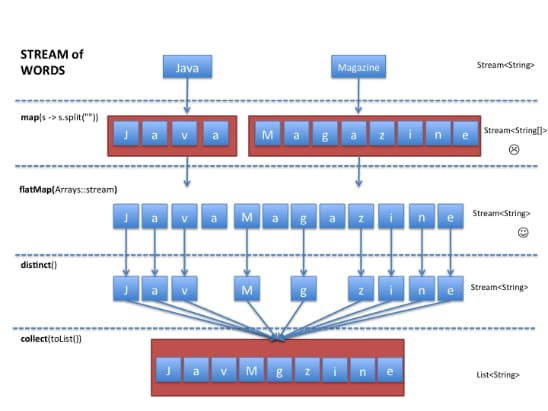Figure 2

In a nutshell, `flatMap` lets you replace each value of a stream with another stream, and then it concatenates all the generated streams into one single stream.

Note that `flatMap` is a common pattern. You will see it again when dealing with `Optional` or `CompletableFuture`.

### collect Operation

Let’s now look at the `collect` method in more detail. The operations you saw in the first part of this series were either returning another stream (that is, they were intermediate operations) or returning a value, such as a `boolean`, an `int`, or an `Optional` object (that is, they were terminal operations).

The `collect` method is a terminal operation, but it is a bit different because you used it to transform a stream into a list. For example, to get a list of the IDs for all the expensive transactions, you can use the code shown in Listing 8.

```import static java.util.stream.Collectors.*;
List<Integer> expensiveTransactionsIds =
transactions.stream()
.filter(t -> t.getValue() > 1000)
.map(Transaction::getId)
.collect(toList());
```

Listing 8

The argument passed to `collect` is an object of type `java .util.stream.Collector`. What does a `Collector` object do? It essentially describes a recipe for accumulating the elements of a stream into a final result. The factory method `Collectors.toList()` used earlier returns a `Collector` describing how to accumulate a stream into a list. However, there are many similar built-in `Collectors` available.

Collecting a stream into other collections. For example, using `toSet()` you can convert a stream into a `Set`, which will remove duplicate elements. The code in Listing 9 shows how to generate the set of only the cities that have expensive transactions. (Note: In all future examples, we assume that the factory methods of the `Collectors` class are statically imported using `import static java.util.stream.Collectors.*`.)

```Set<String> cities =
transactions.stream()
.filter(t -> t.getValue() > 1000)
.map(Transaction::getCity)
.collect(toSet());
```

Listing 9

Note that there are no guarantees about what type of `Set` is returned. However, using `toCollection()` you can have more control. For example, you can ask for a `HashSet` by passing a constructor reference to it (see Listing 10).

```Set<String> cities =
transactions.stream()
.filter(t -> t.getValue() > 1000)
.map(Transaction::getCity)
.collect(toCollection(HashSet::new));
```

Listing 10

However, that’s not all you can do with `collect` and collectors. It is actually a very tiny part of what you can do. Here are some examples of what you can express:

• Grouping a list of transactions by currency to the sum of the values of all transactions with that currency (returning a `Map<Currency, Integer>`)
• Partitioning a list of transactions into two groups: expensive and not expensive (returning a `Map<Boolean, List<Transaction>>`)
• Creating multilevel groupings, such as grouping transactions by cities and then further categorizing by whether they are expensive or not (returning a `Map<String, Map<Boolean, List<Transaction>>>`)

Excited? Great. Let’s see how you can express these queries using the Stream API and collectors. We first start with a simple example that “summarizes” a stream: calculating the average, the maximum, and the minimum of a stream. We then look at how to express simple groupings, and finally we see how to combine collectors together to create powerful queries, such as multilevel groupings.

Summarizing. Let’s warm up with some simple examples. You saw in the previous article how to calculate the number of elements, the maximum, the minimum, and the average of a stream using the `reduce` operation and using primitive streams. There are predefined collectors that let you do just that as well. For example, you can use `counting()` to count the number of items, as shown in Listing 11.

```long howManyTransactions =
transactions.stream().collect(counting());
```

Listing 11

You can use `summing Double()`, `summingInt()`, and `summingLong()` to sum the values of a `Double`, an `Int`, or a `Long` property of the elements in a stream. In Listing 12, we calculate the total value of all transactions.

```int totalValue = transactions.stream().collect(
summingInt(Transaction::getValue));
```

Listing 12

Similarly, you can use `averaging Double()`, `averagingInt()`, and `averagingLong()` to calculate the average, as shown in Listing 13

```double average = transactions.stream().collect(
averagingInt(Transaction::getValue));
```

Listing 13

In addition, by using `maxBy()` and `minBy()` you can calculate the maximum and minimum element of a stream. However, there’s a catch: you need to define an order for the elements of a stream to be able to compare them. This is why `maxBy` and `minBy` take a `Comparator` object as an argument, as illustrated in Figure 3.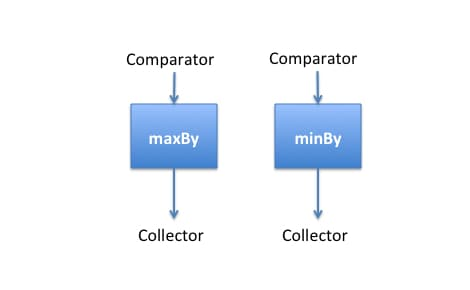Figure 3

In the example in Listing 14, we use the static method `comparing()`, which generates a `Comparator` object from a function passed as an argument. The function is used to extract a comparable key from the element of a stream. In this case, we find the highest transaction by using the value of a transaction as a comparison key.

```Optional<Transaction> highestTransaction =
transactions.stream()
.collect(maxBy(comparing(Transaction::getValue)));
```

Listing 14

There’s also a `reducing()` collector that lets you combine all elements in a stream by repetitively applying an operation until a result is produced. It is conceptually similar to the `reduce()` method you saw previously. For example, Listing 15 shows an alternative way to calculate the sum of all transactions using `reducing()`.

```int totalValue = transactions.stream().collect(reducing(
0, Transaction::getValue, Integer::sum));
```

Listing 15

`reducing()` takes three arguments:

• An initial value (it is returned if the stream is empty); in this case, it is `0`.
• A function to apply to each element of a stream; in this case, we extract the value of each transaction.
• An operation to combine two values produced by the extracting function; in this case, we just add up the values.

You might say, “Wait a minute; I can already do that with other stream methods, such as `reduce()`, `max()`, and `min()`, so why are you showing me this?” You will see later that we can combine collectors to build more-complex queries (for example, grouping plus averaging), so it is handy to know about these built-in collectors as well.

Grouping. A common database query is to group data using a property. For example, you might want to group a list of transactions by currency. Expressing such a query using explicit iteration is somewhat painful, as you can see in the code in Listing 16.

```Map<Currency, List<Transaction>> transactionsByCurrencies =
new HashMap< >();
for(Transaction transaction : transactions) {
Currency currency = transaction.getCurrency();
List<Transaction> transactionsForCurrency =
transactionsByCurrencies.get(currency);
if (transactionsForCurrency == null) {
transactionsForCurrency = new ArrayList<>();
transactionsByCurrencies.put(
currency, transactionsForCurrency);
}
}
```

Listing 16

You need to first create a `Map` where the transactions will be accumulated. Then you need to iterate the list of transactions and extract the currency for each transaction. Before adding the transaction in as a value in the `Map`, you need to check whether a list has been created, and so on. It is a shame, because fundamentally all we want to do is “group the transactions by currency.” Why does it have to involve so much code? Good news: there’s a collector called `groupingBy()` that lets us express such use cases in a concise way. We can express the same query as shown in Listing 17, and now the code reads closer to the problem statement.

```Map<Currency, List<Transaction>> transactionsByCurrencies =
transactions.stream().collect(groupingBy(
Transaction::getCurrency));
```

Listing 17

The `groupingBy()` factory method takes as an argument a function for extracting the key used to classify the transactions. We call it a classification function. In this case, we pass a method reference, `Transaction::getCurrency`, to group the transaction by currency. Figure 4 illustrates the grouping operation.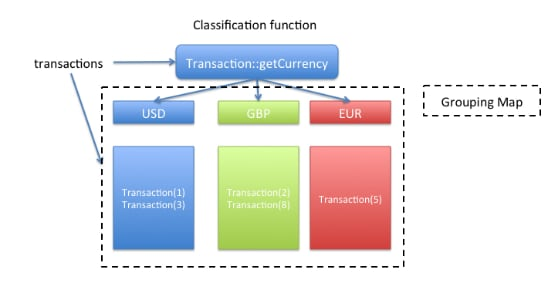Figure 4

Partitioning. There’s another factory method called `partitioningBy()` that can be viewed as a special case of `groupingBy()`. It takes a predicate as an argument (that is, a function that returns a `boolean`) and groups the elements of a stream according to whether or not they match that predicate. In other words, partitioning a stream of transactions organizes the transactions into a `Map<Boolean, List<Transaction>>`. For example, if you want to group the transactions into two lists—cheap and expensive—you could use the `partitioningBy` collector as shown in Listing 18, where the lambda `t -> t.getValue() > 1000` is a predicate for classifying cheap and expensive transactions.

```Map<Boolean, List<Transaction>> partitionedTransactions =
transactions.stream().collect(partitioningBy(
t -> t.getValue() > 1000));
```

Listing 18

Composing collectors. If you are familiar with SQL, you might know that you can combine GROUP BY with functions such as COUNT() and SUM() to group transactions by currency and by their sum. So, can we do something similar with the Stream API? Yes. Actually, there’s an overloaded version of `groupingBy()` that takes another collector object as a second argument. This additional collector is used to define how to accumulate all the elements that were associated with a key using the `groupingBy` collector.

OK, this sounds a bit abstract, so let’s look at a simple example. We would like to generate a `Map` of cities according to the sum of all transactions for each city (see Listing 19). Here, we tell `groupingBy` to use the method `getCity()` as a classification function. As a result, the keys of the resulting `Map` will be cities. We would normally expect a `List<Transaction>` back as the value for each key of the `Map` using the basic `groupingBy`.

```Map<String, Integer> cityToSum =
transactions.stream().collect(groupingBy(
Transaction::getCity, summingInt(Transaction::getValue)));
```

Listing 19

However, we are passing an additional collector, `summingInt()`, which sums all the values of the transactions associated with a city. As a result, we get a `Map<String, Integer>` that maps each city with the total value of all transactions for that city. Cool, isn’t it? Think about it: the basic version of `groupingBy (Transaction::getCity)` is actually just shorthand for writing `groupingBy (Transaction::getCity, toList())`.

Let’s look at another example. How about if you want to generate a `Map` of the highest-valued transaction for each city? You might have guessed that we can reuse the `maxBy` collector we defined earlier, as shown in Listing 20.

```Map<String, Optional<Transaction>> cityToHighestTransaction =
transactions.stream().collect(groupingBy(
Transaction::getCity, maxBy(comparing(Transaction::getValue))));
```

Listing 20

You can see that the Stream API is really expressive; we are now building some really interesting queries that can be written concisely. Can you imagine going back to processing a collection iteratively?

Let’s look at a more-complicated example to finish. You saw that `groupingBy` can take another collector object as an argument to accumulate the elements according to a further classification. Because `groupingBy` is a collector itself, we can create multilevel groupings by passing another `groupingBy` collector that defines a second criterion by which to classify the stream’s elements.

In the code in Listing 21, we group the transactions by city, and then we further group the transactions by the currency of transactions in each city to get the average transaction value for that currency. Figure 5 illustrates the mechanism.

```Map<String, Map<Currency, Double>> cityByCurrencyToAverage =
transactions.stream().collect(groupingBy(Transaction::getCity,
groupingBy(Transaction::getCurrency,
averagingInt(Transaction::getValue))));
```

Listing 21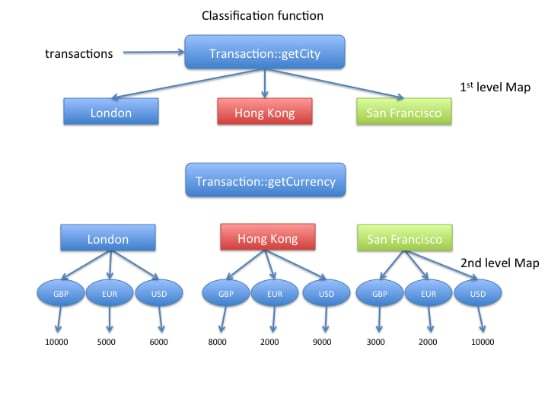Figure 5

Creating your own collector. All the collectors we showed so far implement the interface `java.util.stream .Collector`. This means that you can implement your own collectors to define “customized” reduction operations. However, this subject could easily fit into another article, so we won’t discuss it here.Java 8 in Action: Lambdas, Streams, and functional-style programming

### Conclusion

In this article, we’ve explored two advanced operations of the Stream API: `flatMap` and `collect`. They are tools that you can add to your arsenal for expressing rich data processing queries.

In particular, you’ve seen that the `collect` method can be used to express summarizing, grouping, and partitioning operations. In addition, these operations can be combined to build even richer queries, such as “produce a two-level `Map` of the average transaction value for each currency in each city.”

However, this article didn’t investigate all the built-in collectors that are available. We invite you to take a look at the `Collectors` class and try out other collectors, such as `mapping()`, `joining()`, and `collecting AndThen()`, which you might find useful.Raoul-Gabriel Urma
is currently completing a PhD in computer science at the University of Cambridge, where he does research in programming languages. In addition, he is an author of Java 8 in Action: Lambdas, Streams, and functional-style programming (Manning, 2014).